# Background

The goal of this project is to generate Gaussian samples in 2-D from uniform samples, the latter of which can be readily generated using built-in random number generators in most computer languages.

In part 1 of the project, the inverse transform sampling was used to convert each uniform sample into respective x and y coordinates of our Gaussian samples, which are themselves independent standard normal (having mean of 0 and standard deviation of 1):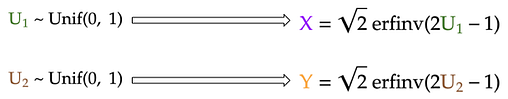Generate x and y coordinates from uniform samples (U₁ and U₂) using inverse transform sampling

However, this method uses the inverse cumulative distribution function (CDF) of the Gaussian distribution, which is not well-defined. Therefore, we approximated this function using the simple Taylor series. However, this only samples accurately near the Gaussian mean, and under-samples more extreme values at both ends of the distribution.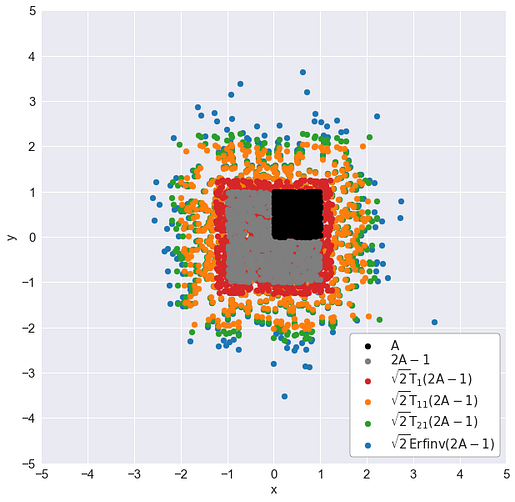A: left-side area (uniformly sampled). Tᵢ: the iᵗʰ-degree Taylor series approximation of the inverse Gaussian CDF: √2 Erfinv(2A-1)

In part 2 of the project, we used the Box-Muller transform, a more direct method to transform the uniform samples into Gaussian ones. The implementation of the algorithm is quite simple, as seen below, but its derivation requires some clever change of variables: instead of sampling Gaussian x and y coordinates for each point, we will sample a uniformly distributed angle (from 0 to 2π) and an exponentially-distributed random variable that represents half of a squared distance of the sample to the origin.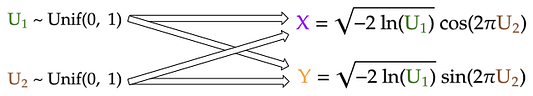Generate x and y coordinates from uniform samples (U₁ and U₂) using Box-Muller transform

In this part of the project, I will present an even simpler method than the above two methods. Even better, this method is one that every statistics students are already familiar with.

# The Central Limit Theorem

It turns out, we can rely on the most fundamental principle of statistics to help us generate Gaussian samples: the central limit theorem. In very simple terms, the central limit theorem states that:

Given n independent random samples from the same distribution, their sum will converge to a Gaussian distribution as n gets large.

Therefore, to generate a Gaussian sample, we can just generate many independent uniform samples and add them together! We then repeat this routine to generate the next standard Gaussian sample until we get enough samples for our x-coordinates. Finally, we just repeat the same steps to generate the y coordinates.

Note that this method will work even if the samples that we start with are not uniform — they are Poisson-distributed, for example. This is because the central limit theorem holds for virtually all probability distributions *cough let’s not talk about the Cauchy distribution cough*.

# Generate Gaussian samples by central limit theorem

To generate, say, 1000 Gaussian sums (`n_sums = 1000`) where each is the sum of 100 uniform samples (`n_additions = 100`):

```n_additions = 100
n_points = 1000# 0. Initialize random number generator
rng = np.random.RandomState(seed=24) # 1. Generate matrix of uniform samples
uniform_matrix = rng.uniform(size=(n_additions, n_points))# 2. Sum uniform elements down each column to get all Gaussian sums
gaussians = uniform_matrix.sum(axis=0)```

We can apply the above method to generate Gaussian samples for each coordinate, using different random number generators for x and for y to ensure that the coordinates are independent of each other. Visualizing the intermediate sums after each addition, we see that:

# Background

In part 1 of this project, I’ve shown how to generate Gaussian samples using the common technique of inversion sampling:

The bad news: the Gaussian inverse CDF is not well-defined, so we have to approximate that function, and the simple Taylor series was used. However, this only samples accurately near the Gaussian mean, and under-samples more extreme values at both ends of the distribution.A: left-side area (uniformly sampled). Tᵢ: the iᵗʰ-degree Taylor series approximation of the inverse Gaussian CDF: √2 Erfinv(2A-1)

Therefore, in this part of the project, we will investigate a more “direct” sampling method that does not depend on the approximation of the Gaussian inverse CDF. This method is called the Box-Muller transform, after the two mathematicians who invented the method in 1958: the British George E. P. Box and the American Mervin E. Muller.Left: George E. P. Box (1919–2013). Right: Mervin E. Muller (1928–2018)

# How does the Box-Muller transform work?

For this project, my goal is to generate Gaussian samples in two dimensions i.e. generating samples whose x and y coordinates are independent standard normals (Gaussian with zero mean and standard deviation of 1). In part 1, I used the inverse Gaussian CDF to generate separately the x and y coordinates from their respective uniform samples (U₁ and U₂):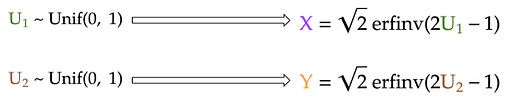Generate x and y coordinates from uniform samples (U₁ and U₂) using inverse transform sampling

For the Box-Muller transform, I will also start with the same two uniform samples. However, I will transform these uniform samples into the x and y coordinates using much simpler formulas: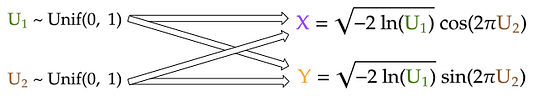Generate x and y coordinates from uniform samples (U₁ and U₂) using Box-Muller transform

Despite the strong coupling between U₁ and U₂ in each of the two formulas above, the generated x and y coordinates, which are both standard Gaussians, are still surprisingly independent from each other! In the derivation of the Box-Muller transform that follows, I will demonstrate why this is indeed this case.

# Derivation of Box-Muller transform

We know that for any two independent random variables x and y, the joint probability density f(x, y) is simply the product of the individual density functions: f(x) and f(y). Furthermore, the Pythagoras theorem allows us to combine the x² and y² terms in each of the Gaussian density functions. This results in the -r²/2 term in the exponential of the joint distribution, where r is the distance from the origin to the 2-D Gaussian sample.

To make it simpler, we then define a variable s that is equal to r²/2. In other words, s is simply half of the squared distance from the origin of our Gaussian sample. Written this way, the joint PDF is simply the product of a constant (1/2π) and an exponential (e⁻ˢ).

# Background

Gaussian sampling — that is, generating samples from a Gaussian distribution — plays an important role in many cutting-edge fields of data science, such as Gaussian processvariational autoencoder, or generative adversarial network. As a result, you often see functions like tf.random.normal in their tutorials.

But, deep down, how does computer know how to generate Gaussian samples? This series of blog posts will show 3 different ways that we can program our computer (via Python) to do so. You will also see how R and Python generate Gaussian samples using modified versions of some of these methods.

# Starting point: the uniform number generator

Of course, we can’t generate Gaussian samples from thin air. Instead, we start with a random number generator that exists in almost all programming languages: the uniform random number generator. It generates a random number that could take any value between 0 and 1. For Python, the numpy.random module uses the Mersenne twister to generate a uniformly-distributed float that is in the interval [0.0, 1.0).

Since Gaussians are better visualized in 2 dimensions — we are all familiar with the Gaussian “blob” in the xy-plane — I will demonstrate the 3 sampling methods in 2-D, especially since one of the methods do generate Gaussians in two dimensions at the same time.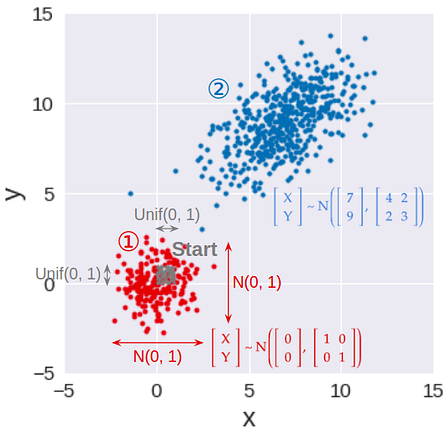Step 1: Generate standard Gaussian samples in 2-D. Step 2: Transform standard Gaussian samples to have given means, variances, and covariance between x and y

As a result, this series is broken down into 3 parts (see accompanying image):

# Method 1: Inverse transform sampling

This is the most basic, yet most common, way to convert a uniform random sample into a random sample of any distribution, including Gaussian. This method works by applying the inverse function of the Gaussian CDF (cumulative distribution function) to transform a uniform sample into a Gaussian sample.

To make sure that the Gaussian samples for the x- and y-coordinates are independent, we can use two different uniform samples, one for x (U₁), and one for y (U₂). These two uniform samples can be generated using two different random number generators (two different `RandomState` initialized by different seeds, for example) so that they are independent in the first place.